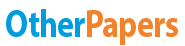Red Brand Canners

Essay by   •  October 15, 2011  •  Essay  •  542 Words (3 Pages)  •  1,174 Views

Essay Preview: Red Brand Canners

Report this essay
Page 1 of 3

Red Brand Canners

Problem 1:

Using 3,000,000 tomatoes for production (without extra tomatoes)

Decision variables

a1 = Amount of A grade tomatoes in Whole tomatoes

b1 = = Amount of B grade tomatoes in Whole tomatoes

a2 = Amount of A grade tomatoes in tomatoes juice

b2 = Amount of B grade tomatoes in tomatoes juice

b3 = Amount of B grade tomatoes in tomato paste

Resource Constraints:

C1 a1-3b1 >= 0

C2 3a2-b2 >= 0

C3 a1+b1 <= 14400000

C4 a2+b2 <= 1000000

C5 b3 <= 2000000

C6 a1+a2 <= 600000

C7 b1+b2+b3 <= 2400000

a1, a2, b1, b2, b3 >= 0

Objective Function:

Z = 0.0822 a1 + 0.0822 b1 + 0.066 a2 + 0.066 b2 + 0.074 b3

Maximize Z

Objective Cell ()

Cell Name Original Value Final Value

\$O\$13 <= 225340 225340

a1 b1 a2 b2 b3

525000 175000 75000 225000 2000000

Problem 2:

Using 3,000,000 tomatoes for production and also purchase extra tomatoes

Decision variables

a1 = Amount of A grade tomatoes in Whole tomatoes

b1 = = Amount of B grade tomatoes in Whole tomatoes

a2 = Amount of A grade tomatoes in tomatoes juice

b2 = Amount of B grade tomatoes in tomatoes juice

b3 = Amount of B grade tomatoes in tomato paste

Resource Constraints:

C1 a1-3b1 >= 0

C2 3a2-b2 >= 0

C3 a1+b1 <= 14400000

C4 a2+b2 <= 1000000

C5 b3 <= 2000000

C6 a1+a2 <= 680000

C7 b1+b2+b3 <= 2400000

a1, a2, b1, b2, b3 >= 0

Objective Function:

Z = -0.028 a1 + 0.0822 b1 - 0.019 a2 + 0.066 b2 + 0.074 b3 + 51000 -180000

Maximize Z

Z= 46875.5

a1 b1 a2 b2 b3

525000 175000 75000 225000 2000000

esource Constraints:

C1 a1-3b1 >= 0

C2 3a2-b2 >= 0

C3 a1+b1 <= 14400000

C4 a2+b2 <= 1000000

C5 b3 <= 2000000

C6 a1+a2 <= 600000

C7 b1+b2+b3 <= 2400000

esource Constraints:

C1 a1-3b1 >= 0

...

...